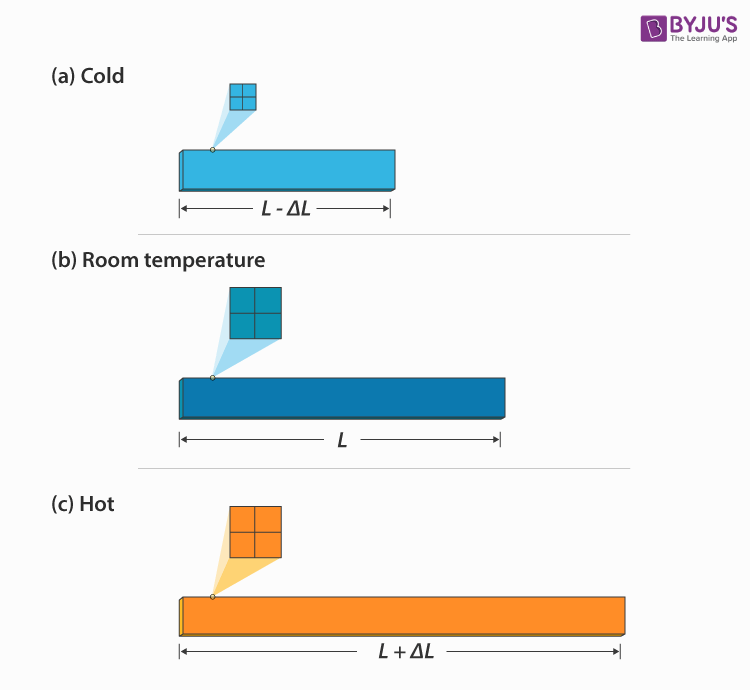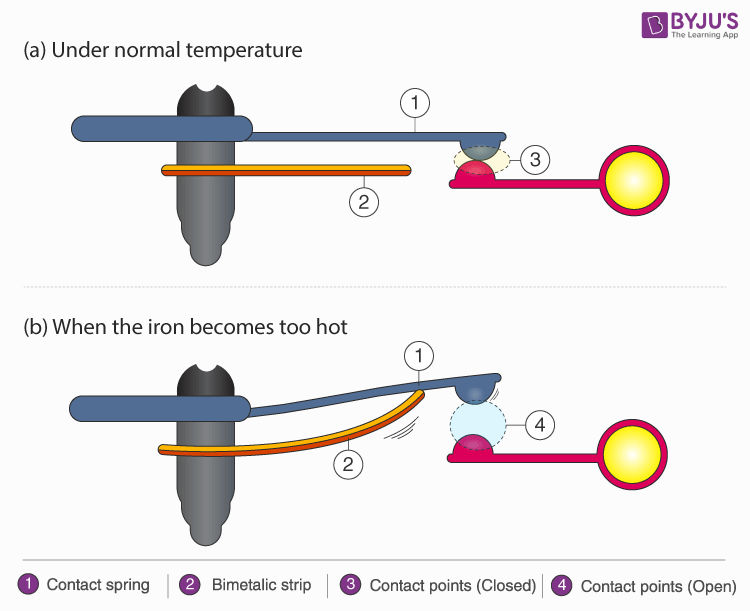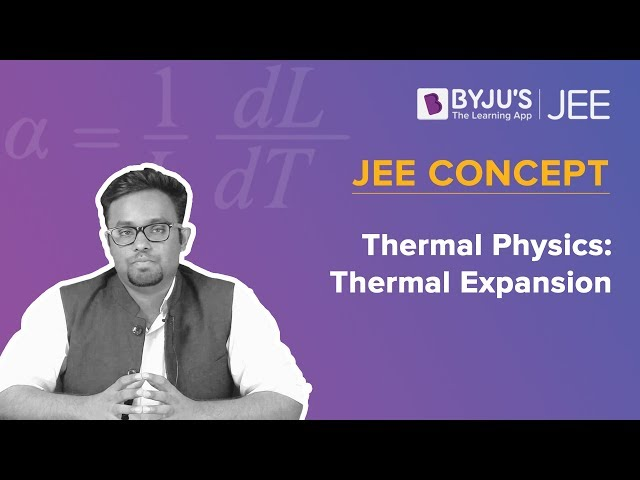# Thermal Stress

Thermal stress is the stress produced by any change in the temperature of the material. Thermal stress is induced in a body when the temperature of the body is raised or lowered and the body is not allowed to expand or contract freely. Thermal stress includes both heat and cold stress. In this article, we will learn about the thermal stress definition, formula and applications in detail.

## What is Thermal Stress?

Stress is the force acting per unit area. The force can be of any form. When the applied force is in the form of temperature the resultant stress is called Thermal stress. It is observed when an object expands or contracts due to a change in temperature. Thus, we can define Thermal stress as:

“Stress caused due to the change in temperature”

It is measured using feet or meters. The effect of temperature on conductors is vital. The thermal conductors expand on heating and contract on cooling.## Thermal Stress Formula

Consider a thermal conducting rod, on heating, the rod expands. The change in length will be directly proportional to the amount of heat supplied and the coefficient of thermal expansion. Thus, we can mathematically write thermal stress as:

 $$\begin{array}{l}\delta _{T}=L\alpha \left ( T_{f} -T_{i}\right )\end{array}$$ $$\begin{array}{l}\delta _{T}=L\alpha \Delta T\end{array}$$

Where,

• L is the length in metres
• Ti is the initial temperature in °C
• Tf is the final temperature in °C
• ΔT=Tf-Ti is the change in temperature in °C
• α is coefficient of thermal expansion

(Coefficient of thermal expansion defines the rate at which shape/physical dimensions of object changes according to change in temperature. It varies from one material to another)

## Applications of Thermal Stress

Thermal stress has got plenty of applications. Some of them are listed below:

### Thermostat

A thermostat is the most commonly known application of Thermal stress. As the word implies “Thermo” means temperature and “stat” means static, Thermostat is a device used to regulate/maintain a constant temperature. They are widely used in the construction of various electronic devices, ranging from air conditioners to iron box.

### Iron box: Bimetallic strips

The thermostat used in an iron box contains a “bimetallic strip”. As the very name suggests, bimetallic strips mean, “a metallic strip made up of two metals of the different coefficient of thermal expansion bonded together”. Thus on supplying the same amount of heat two metals expand at a different rate.

## Working:At room temperature, the contact points continue to be in physical contact with bimetallic strips. However, on heating, the moment temperature crosses the threshold limit. The bimetallic strip starts bending. Bending will be higher towards the metal strip with a lower coefficient of thermal expansion (𝛼). As a result, the physical connection to the contact point is ceased. The circuit opens and there will be no further flow of electricity/current.

The circuit remains open until the bimetallic strip cools down and attains its original shape. Once the strip acquires its original shape, the circuit closed, and the current flows. The same cycle will be repeated until the power supply is turned off.

Now you clearly got to know why your iron box turns on and off on its own! There is no intelligence involved. It is just a simple application of thermal stress.

You may also want to check out these topics given below!

## Watch the video and learn how linear expansion varies with temperature.## Frequently Asked Questions – FAQs

### What is meant by thermal stress?

Stress is caused by variations in temperature.

### What is a thermostat?

A thermostat is an instrument used to maintain a constant temperature.

### What are the major thermal properties of a material?

Heat capacity, thermal expansion, thermal conductivity and thermal stress.

### What is meant by heat capacity?

It is defined as the quantity of heat needed to vary the temperature of a given object by one degree.

### What is the heat capacity formula?

Q=mc ΔT

Stay tuned with BYJU’S to learn more Physics-concepts with the help of interactive video lessons.

Test your Knowledge on Thermal Stress